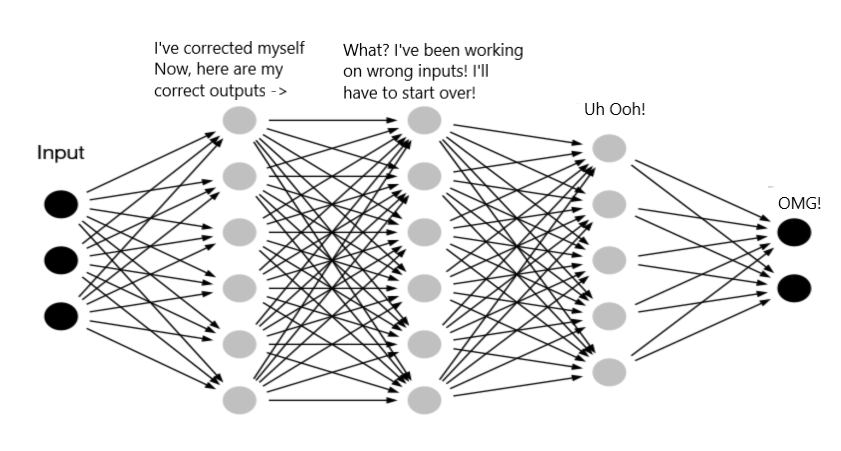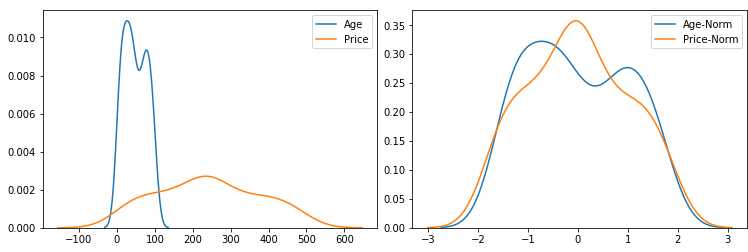# 批量归一化（BatchNorm）加速深度网络训练

1. 靠近输出侧的各层需要不断去重新适应参数更新，学习速度慢。
2. 每层的更新都会影响到其他层，为了训好模型，我们需要非常谨慎地去设定学习率、初始化权重、以及尽可能细致的参数更新策略。Internal Covariate Shift

## 批量归一化的数学表示年龄和价格特征，单位不同，作为模型的输入，需要先进行归一化

\begin{aligned} \hat{\boldsymbol{\mu}}_\mathcal{B} &= \frac{1}{m}\sum_{i = 1}^{m} \boldsymbol{x^{(i)}},\\ \hat{\boldsymbol{\sigma}}_\mathcal{B}^2 &= \frac{1}{m}\sum_{i = 1}^{m} (\boldsymbol{x^{(i)}} - \hat{\boldsymbol{\mu}}_{\mathcal{B}})^2 + \epsilon.\end{aligned} 其中，$m$为批次中的样本数量。另外，$\epsilon > 0$是一个很小的常数，因为Batch Normalization在计算过程中，$\hat{\boldsymbol{\sigma}}_\mathcal{B}$在分母上，需要保证分母大于0。

## 全连接和卷积的批量归一化

### 卷积层的批量归一化

Batch Normalization在一定程度上解决了计算不稳定的问题，可以加速训练的过程，原始论文提到：

We apply Batch Normalization to the best-performing ImageNet classification network, and show that we can match its performance using only 7% of the training steps, and can further exceed its accuracy by a substantial margin.

## 框架实现

1. 深度学习中 Batch Normalization为什么效果好？
2. Ioffe, S., & Szegedy, C. (2015). Batch normalization: accelerating deep network training by reducing internal covariate shift.
3. Batch Normalization — Speed up Neural Network Training
4. http://d2l.ai/chapter_convolutional-modern/batch-norm.html

5. 详解深度学习中的Normalization，BN/LN/WN